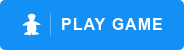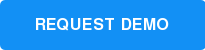# Play ST Math Games

### Play sample puzzles from various stages of the highly scaffolded ST Math curriculum

K-2
Push Box

Students are introduced to the concept of addition from 1 to 10 by selecting the number of blocks needed to get JiJi, the penguin, to the height of the platform. By visually introducing addition as stacking blocks and subtraction as holes in the ground, students see how addition and subtraction are the inverse of each other.

As the puzzles transition, students solve multi-step puzzles to determine the height JiJi will end up at.K-5
Upright JiJi

### Topic: Spatial Reasoning

Can you rotate JiJi, the penguin, into an upright position?

This task specifically targets students spatial temporal reasoning ability as they manipulate 3D JiJi into an upright position.

Upright JiJi develops pre-algebraic thinking and reasoning, as students select the correct set of operators to transform the input into the output.1-2
Number Line Trap

### Topic: Estimation on the Number Line

Build number sense by estimating the location of a dot on the number line.

As the numbers get larger, students must extend their understanding into the 100's and 1,000's, leveraging their understanding of place value. Asking students to estimate rather than select the exact value helps to build a conceptual framework of the model.3-5
How Many Legs?

### Topic: Multiplication, Division

How many shoes are needed for all the animals feet?

Students develop their understanding of equal groups multiplication, through 40, by selecting the number of shoes required by the group of animals.

In later levels, the game uses the same model to represent division and multi-step problem situations, building a robust and interconnected schema.3-5
Fair Sharing

### Topic: Division

Drag blocks onto creatures in order to divide a set of blocks into equal piles. Through this task, students model the task of "fair sharing" division.

Students will slowly transition to answering "fair sharing" questions up to 40 in a more symbolic representation, building their division schema on a conceptual foundation.3-5
JiJi Cycle

### Topic: Fractions

What is the relationship between fraction pies and the number line?

In this creative model, students estimate how far a set of fraction wedges will roll JiJi onto the number line.

As the level progresses, students must weigh how the impact of unit-fractions, fraction addition, and subtraction will alter the solution.